Function Repository Resource:

# DeBruijnNewmanH

Compute the values of the function from which the de Bruijn–Newman constant is defined

Contributed by: Riccardo Gatti
 ResourceFunction["DeBruijnNewmanH"][z] computes the value H0(z) where H is the function from which the de Bruijn–Newman constant Λ is defined.

## Details and Options

For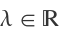and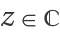, the function is defined as follows: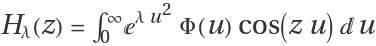where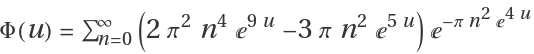is the super-exponentially decaying function.
Hλ(z) is symmetrical with respect of z=0, hence Hλ(-z)=Hλ(z).
H0(z) is related to the RiemannXi function via the identity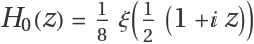, where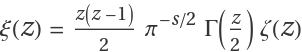. Hence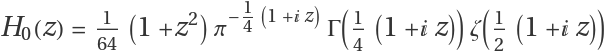and from definition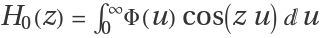.

## Examples

### Basic Examples (3)

Compute the value of H0(2+5ⅈ):

 In:=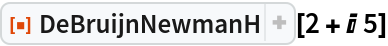Out=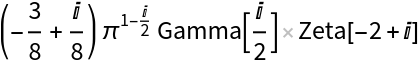Plot of the real part of the complex function H0(x+100ⅈ):

 In:=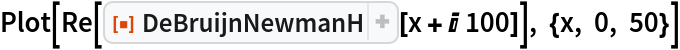Out=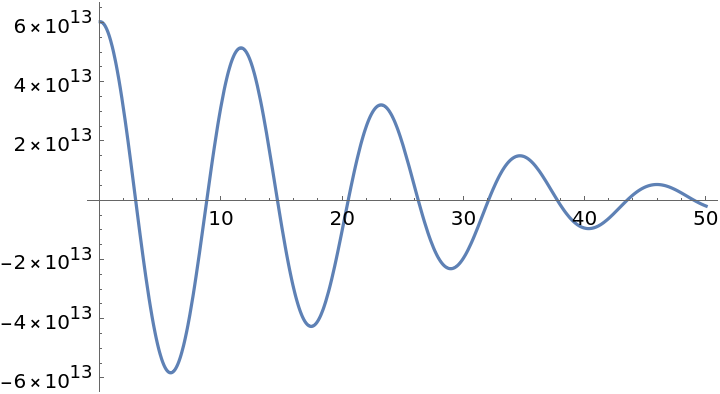Calculate the value of H0(z) using both an integral definition and the relation with Riemann ζ function. As WorkingPrecision gets larger, the difference between two definitions approaches 0 and the computation could take a few minutes:

 In:=Out=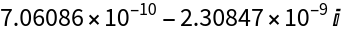### Scope (1)

If the Riemann hypothesis is correct, then all zeros of H0(z) are real. We check for a specific zero z around 20 that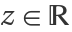:

 In:=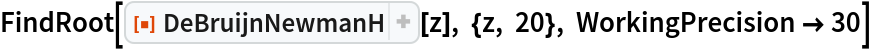Out=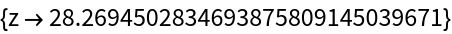Riccardo Gatti

## Version History

• 1.0.0 – 20 January 2021